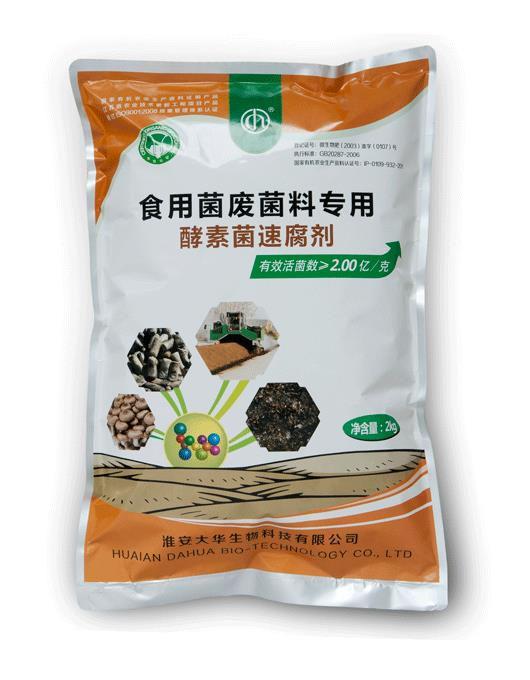• 水产专用
• 大量元素水溶肥料
• 含腐殖酸水溶肥料
• 含氨基酸水溶肥料
• 发酵菌剂
• 沼气发酵菌剂
• 肥水专用
• 净水剂
• 发酵增产剂
• 生物反应堆菌剂
• 秸秆饲料发酵剂
• 酵素菌速腐剂
• 废菌料发酵剂
• 育苗基质
• 水稻育苗基质
• 蓝莓栽培基质
• 蔬菜育苗基质
• 生物有机肥
• 有机肥料
• 生物有机肥
• 食用菌专用
• 发酵增产剂
• 菇宝乐
• 联系我们
• 产品分类
• 发酵菌剂
• 生物反应堆菌剂
• 沼气发酵菌剂
• 肥水专用
• 发酵增产剂
• 秸秆饲料发酵剂
• 废菌料发酵剂
• 酵素菌速腐剂
• 净水剂
• 水溶肥料
• 大量元素水溶肥料
• 含腐殖酸水溶肥料
• 含氨基酸水溶肥料
• 育苗基质
• 蓝莓栽培基质
• 蔬菜育苗基质
• 水稻育苗基质
• 生物有机肥
• 有机肥料
• 生物有机肥
• 水产制剂
• 水产专用
• 食用菌专用
• 菇宝乐
• 发酵增产剂
• 园艺资材
• 有机肥料
• 水稻育苗基质
• 花盆
• 含氨基酸水溶肥料
• 菇宝乐
• 发酵增产剂
• 土壤修复剂
• 土壤调理剂
• 产品分类
• 发酵菌剂
• 水溶肥料
• 育苗基质
• 生物有机肥
• 食用菌专用
• 园艺资材
• 土壤调理剂
• 土壤修复剂
• 水产制剂
• 产品分类
• 土壤调理剂
• 土壤修复剂
• 水产制剂
• 水产专用
• 育苗基质
• 蓝莓栽培基质
• 蔬菜育苗基质
• 水稻育苗基质
• 发酵菌剂
• 发酵增产剂
• 肥水专用
• 沼气发酵菌剂
• 净水剂
• 生物反应堆菌剂
• 秸秆饲料发酵剂
• 废菌料发酵剂
• 酵素菌速腐剂
• 水溶肥料
• 大量元素水溶肥料
• 含氨基酸水溶肥料
• 含腐殖酸水溶肥料
• 生物有机肥
• 有机肥料
• 生物有机肥
• 食用菌专用
• 菇宝乐
• 发酵增产剂
• 园艺资材
• 菇宝乐
• 发酵增产剂
• 花盆
• 水稻育苗基质
• 含氨基酸水溶肥料
• 有机肥料
• 产品分类
• 发酵菌剂
• 发酵增产剂
• 沼气发酵菌剂
• 净水剂
• 生物反应堆菌剂
• 秸秆饲料发酵剂
• 废菌料发酵剂
• 酵素菌速腐剂
• 肥水专用
• 水溶肥料
• 大量元素水溶肥料
• 含腐殖酸水溶肥料
• 含氨基酸水溶肥料
• 育苗基质
• 蓝莓栽培基质
• 蔬菜育苗基质
• 水稻育苗基质
• 生物有机肥
• 有机肥料
• 生物有机肥
• 土壤调理剂
• 食用菌专用
• 菇宝乐
• 发酵增产剂
• 园艺资材
• 有机肥料
• 水稻育苗基质
• 花盆
• 含氨基酸水溶肥料
• 发酵增产剂
• 菇宝乐
• 土壤修复剂
• 水产制剂
• 水产专用
• 产品分类
• 土壤调理剂
• 土壤修复剂
• 水产制剂
• 水产专用
• 发酵菌剂
• 沼气发酵菌剂
• 肥水专用
• 净水剂
• 发酵增产剂
• 生物反应堆菌剂
• 秸秆饲料发酵剂
• 废菌料发酵剂
• 酵素菌速腐剂
• 水溶肥料
• 含氨基酸水溶肥料
• 含腐殖酸水溶肥料
• 大量元素水溶肥料
• 育苗基质
• 蓝莓栽培基质
• 蔬菜育苗基质
• 水稻育苗基质
• 生物有机肥
• 有机肥料
• 生物有机肥
• 食用菌专用
• 菇宝乐
• 发酵增产剂
• 园艺资材
• 含氨基酸水溶肥料
• 菇宝乐
• 发酵增产剂
• 水稻育苗基质
• 有机肥料
• 花盆
• 网站首页
• 关于我们
• 总经理致辞
• 企业文化
• 组织架构
• 公司概况
• 产品分类
• 土壤调理剂
• 水产制剂
• 水产专用
• 园艺资材
• 有机肥料
• 水稻育苗基质
• 花盆
• 含氨基酸水溶肥料
• 菇宝乐
• 发酵增产剂
• 育苗基质
• 蓝莓栽培基质
• 蔬菜育苗基质
• 水稻育苗基质
• 生物有机肥
• 有机肥料
• 生物有机肥
• 食用菌专用
• 发酵增产剂
• 菇宝乐
• 土壤修复剂
• 水溶肥料
• 大量元素水溶肥料
• 含腐殖酸水溶肥料
• 含氨基酸水溶肥料
• 发酵菌剂
• 废菌料发酵剂
• 酵素菌速腐剂
• 沼气发酵菌剂
• 肥水专用
• 净水剂
• 发酵增产剂
• 生物反应堆菌剂
• 秸秆饲料发酵剂
• 产品分类
• 发酵菌剂
• 沼气发酵菌剂
• 肥水专用
• 净水剂
• 发酵增产剂
• 生物反应堆菌剂
• 秸秆饲料发酵剂
• 废菌料发酵剂
• 酵素菌速腐剂
• 水溶肥料
• 大量元素水溶肥料
• 含腐殖酸水溶肥料
• 含氨基酸水溶肥料
• 育苗基质
• 蓝莓栽培基质
• 蔬菜育苗基质
• 水稻育苗基质
• 生物有机肥
• 有机肥料
• 生物有机肥
• 食用菌专用
• 菇宝乐
• 发酵增产剂
• 园艺资材
• 含氨基酸水溶肥料
• 有机肥料
• 花盆
• 菇宝乐
• 发酵增产剂
• 水稻育苗基质
• 土壤调理剂
• 土壤修复剂
• 水产制剂
• 水产专用
• 产品分类
• 园艺资材
• 有机肥料
• 水稻育苗基质
• 花盆
• 含氨基酸水溶肥料
• 菇宝乐
• 发酵增产剂
• 土壤调理剂
• 水产制剂
• 水产专用
• 土壤修复剂
• 发酵菌剂
• 生物反应堆菌剂
• 沼气发酵菌剂
• 肥水专用
• 发酵增产剂
• 秸秆饲料发酵剂
• 废菌料发酵剂
• 酵素菌速腐剂
• 净水剂
• 食用菌专用
• 菇宝乐
• 发酵增产剂
• 水溶肥料
• 大量元素水溶肥料
• 含腐殖酸水溶肥料
• 含氨基酸水溶肥料
• 育苗基质
• 蓝莓栽培基质
• 蔬菜育苗基质
• 水稻育苗基质
• 生物有机肥
• 生物有机肥
• 有机肥料
• 产品分类
• 食用菌专用
• 菇宝乐
• 发酵增产剂
• 水溶肥料
• 大量元素水溶肥料
• 含腐殖酸水溶肥料
• 含氨基酸水溶肥料
• 育苗基质
• 蓝莓栽培基质
• 蔬菜育苗基质
• 水稻育苗基质
• 生物有机肥
• 生物有机肥
• 有机肥料
• 园艺资材
• 有机肥料
• 水稻育苗基质
• 花盆
• 含氨基酸水溶肥料
• 菇宝乐
• 发酵增产剂
• 土壤调理剂
• 土壤修复剂
• 水产制剂
• 水产专用
• 发酵菌剂
• 废菌料发酵剂
• 酵素菌速腐剂
• 发酵增产剂
• 秸秆饲料发酵剂
• 生物反应堆菌剂
• 沼气发酵菌剂
• 肥水专用
• 净水剂
• 产品分类
• 发酵菌剂
• 沼气发酵菌剂
• 肥水专用
• 生物反应堆菌剂
• 净水剂
• 秸秆饲料发酵剂
• 废菌料发酵剂
• 酵素菌速腐剂
• 发酵增产剂
• 土壤修复剂
• 水溶肥料
• 大量元素水溶肥料
• 含腐殖酸水溶肥料
• 含氨基酸水溶肥料
• 育苗基质
• 蓝莓栽培基质
• 蔬菜育苗基质
• 水稻育苗基质
• 水产制剂
• 水产专用
• 食用菌专用
• 菇宝乐
• 发酵增产剂
• 园艺资材
• 有机肥料
• 水稻育苗基质
• 花盆
• 含氨基酸水溶肥料
• 菇宝乐
• 发酵增产剂
• 土壤调理剂
• 生物有机肥
• 生物有机肥
• 有机肥料
• 产品分类
• 育苗基质
• 蓝莓栽培基质
• 蔬菜育苗基质
• 水稻育苗基质
• 生物有机肥
• 有机肥料
• 生物有机肥
• 食用菌专用
• 菇宝乐
• 发酵增产剂
• 土壤调理剂
• 土壤修复剂
• 水产制剂
• 水产专用
• 园艺资材
• 水稻育苗基质
• 有机肥料
• 花盆
• 含氨基酸水溶肥料
• 菇宝乐
• 发酵增产剂
• 发酵菌剂
• 沼气发酵菌剂
• 肥水专用
• 净水剂
• 发酵增产剂
• 生物反应堆菌剂
• 秸秆饲料发酵剂
• 废菌料发酵剂
• 酵素菌速腐剂
• 水溶肥料
• 含腐殖酸水溶肥料
• 大量元素水溶肥料
• 含氨基酸水溶肥料
• 产品分类
• 土壤调理剂
• 土壤修复剂
• 水产制剂
• 水产专用
• 发酵菌剂
• 沼气发酵菌剂
• 肥水专用
• 净水剂
• 发酵增产剂
• 生物反应堆菌剂
• 秸秆饲料发酵剂
• 废菌料发酵剂
• 酵素菌速腐剂
• 水溶肥料
• 含氨基酸水溶肥料
• 含腐殖酸水溶肥料
• 大量元素水溶肥料
• 育苗基质
• 蓝莓栽培基质
• 蔬菜育苗基质
• 水稻育苗基质
• 生物有机肥
• 有机肥料
• 生物有机肥
• 食用菌专用
• 菇宝乐
• 发酵增产剂
• 园艺资材
• 花盆
• 菇宝乐
• 发酵增产剂
• 水稻育苗基质
• 含氨基酸水溶肥料
• 有机肥料
• ### 废菌料发酵剂产品名称： 废菌料发酵剂

发布日期： 2016-07-26

点击数： 2891

询价：

二维码：简述： 淘宝链接（选中全部链接打开即可）：https://item.taobao.com/item.htm?spm=a1z10.1-c.w4004-15749265087.8.45ee61bbzl9aZX&id=543871872863

### 产品详情

产品说明

食用菌废菌料腐熟剂是采用微生物技术制成的一种好（兼）气性复合微生物制剂，高效、绿色、环保，内含大量有益微生物、活性酶，适用于香菇、木耳、蘑菇、平菇等各种食用菌废菌料的发酵处理，通过快速腐熟、灭菌和转化养分，可将物料发酵成为营养丰富、功能全面的生物有机肥、基质产品，该肥不仅养分全面、无病菌而且含有大量的有益微生物及其代谢产物，是种植绿色、有机食品的优良肥料。本产品已在食用菌行业广泛使用，深受广大食用菌种植户的欢迎和认可。

主要功效

1、复合菌群优势明显，促进废菌料快速分解；

2、发酵能力强，能快速腐熟废菌料中木屑、玉米芯、蔗渣等物质，提高堆肥效率；

3、可提高肥料利用率，增产效果显著；废菌料发酵转化成的生物有机肥料，施到土壤中改善土壤营养状况，增加土壤有机质；

4、发酵腐熟过程中产生的多种代谢产物，可促进农作物生长，改善农产品品质；促进有益微生物增殖，增强作物抗逆性；

技术指标

有效活菌数总量（cfu）≥2.00亿/g

保质期≥24个月   生产日期：见封口

注意事项

密封置阴凉干燥处存放，勿与杀菌剂同时使用。

使用方法

1、废菌料准备先将废菌料粉碎成2-3mm的颗粒，将菌料湿度调整到50%左右，以物料手握成团不滴水为宜。

2、腐熟剂用量按3公斤/吨，即：发酵处理1吨废菌料干料，需投入发酵菌剂3公斤。

3、发酵方法将腐熟剂均匀撒在废菌料上，充分搅拌后，堆成高1.5米、宽2-3米的条型堆垛进行发酵，发酵48小时后堆温升温至50℃以上，可进行翻堆，以后每天翻堆1次，共翻4-5次可达到无害化标准，进行二次发酵后即可使用。或者直接投入到多孔补氧自动发酵箱内，并用无纺布或者草帘等透气的材料覆盖，即可开始发酵，箱内菌料将逐步升温在4天左右即可达到60-70℃，杀灭废料的有害菌和虫卵，10天左右即可转化成黑褐色、松软、无异味、营养全面的生物有机肥。

4、废菌料有机肥使用方法菌料有机肥肥效释放缓慢周期长，应根据不同作物，不同土壤，科学使用，总的使用原则是“重底肥早追肥”，即底肥重施，追肥早施。使用量100-500公斤/亩，以撒施、条施、穴施为主。

版权所有：淮安亚博体育 登录生物科技有限公司
技术支持：仕德伟科技 苏ICP备16009131号